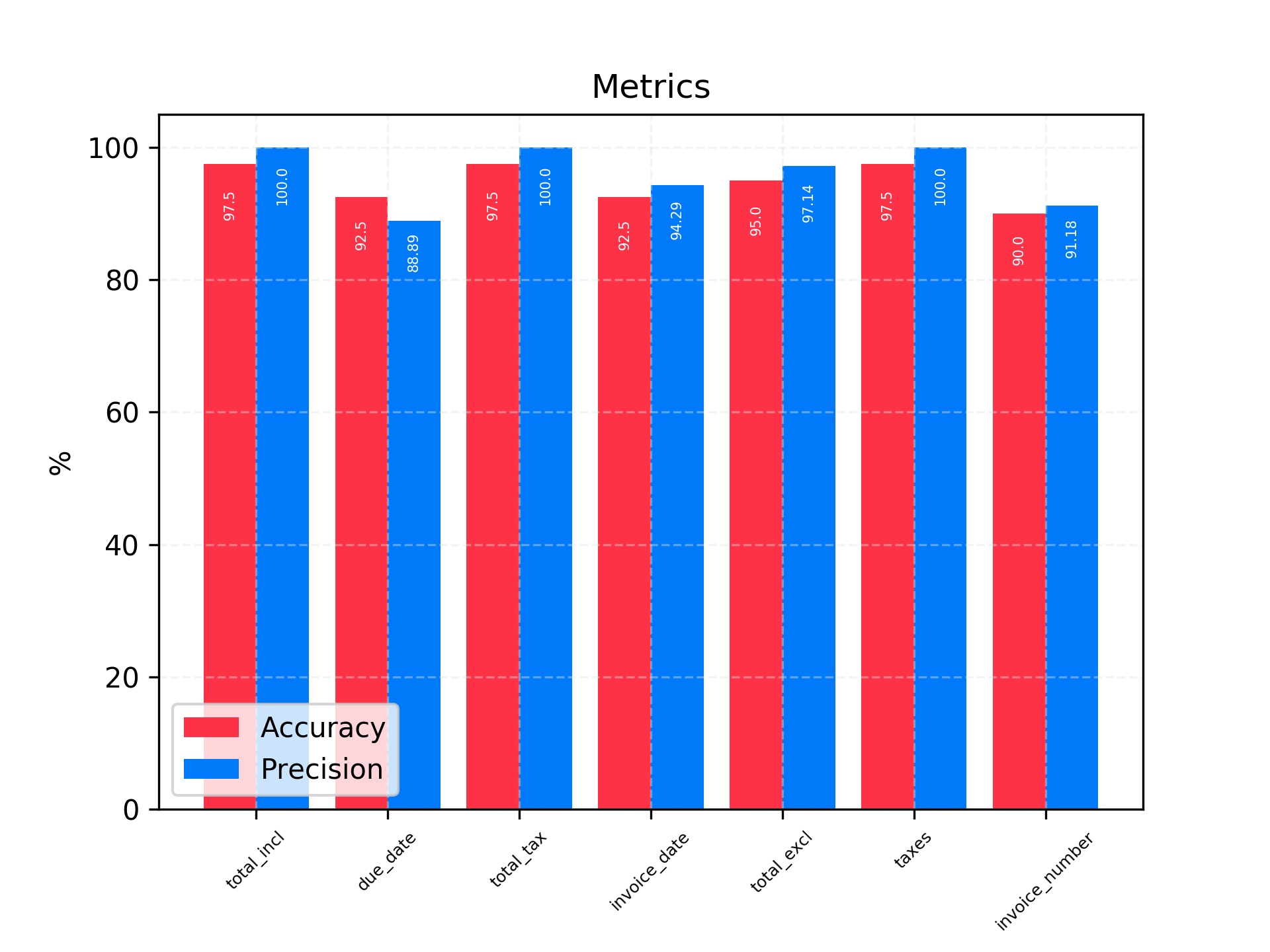# Performances benchmark

In a few steps, you can use the mindee python library to perform benchmarks of invoice extraction perfomances against your test dataset.

1. Perform api calls for each file in your test set
2. Create `mindee.Invoice` objects from your data (csv, json or whatever)
3. Perform the benchmark and analyze the results

## 1. Perform api calls for each file in your test set

Before running the benchmark, we need to collect all the invoice predictions from Mindee API and store them so we can perform the benchmark later without calling the API again.

To do so, assuming that all your files are stored into a "./test_dataset" folder and that we want to save all the responses into a "./mindee_responses" folder, here is the code:

``````from mindee import Client
import os

TEST_DATA_PATH = "./test_dataset"
RESPONSES_PATH = "./mindee_responses"

mindee_client = Client(invoice_token="your_invoices_api_token_here")

# Loop over all your test files
for test_filename in os.listdir(TEST_DATA_PATH):
# Get the current file path
test_file_path = os.path.join(TEST_DATA_PATH, test_filename)

# To make sure we don't stop the process if an error occurs
try:
# Parse the current file
mindee_response = mindee_client.parse_invoice(test_file_path)

# Store the response inside json file to be restored later
# In this example we use the test file name in the json filename to
# be able to retrieve the corresponding file
response_filepath = os.path.join(RESPONSES_PATH, test_filename+".json")
mindee_response.dump(response_filepath)
except Exception as e:
# In case of error, print the filename so you can understand later
# what happened
print(test_filename, e)
``````

## 2. Create `mindee.Invoice` objects from your data (csv, json, whatever...)

The `mindee.Invoice` class contains a `compare()` method that takes as inputs two `mindee.Invoice` objects. Before running our final script, we need now to create a `mindee.Invoice object` containing the true labels for each fields.

We'll use a csv file in this example and the pandas library.

### ground_truth.csv

``````filename,total_incl,total_excl,invoice_date,invoice_number,due_date,taxes
1.jpg,149.5,115,2020-12-01,F0012020,2021-01-01,11.5-10|23-20``````

To construct an Invoice object from this dummy csv example, you can simply do:

``````import pandas as pd
from mindee import Invoice

def invoice_from_csv_row(df_row):
taxes_list = df_row["taxes"].split("|")
return Invoice(
total_incl=df_row["total_incl"],
due_date=df_row["due_date"],
total_excl=df_row["total_excl"],
invoice_date=df_row["invoice_date"],
taxes=[(t.split("-"), t.split("-")) for t in taxes_list],
invoice_number=df_row["invoice_number"]
)

for index, df_row in ground_truth_df.iterrows():
invoice_truth = invoice_from_csv_row(df_row)
print(invoice_truth)
``````

Running this code should print in your console something like this:

``````-----Invoice data-----
Filename: None
Invoice number: F0012020
Total amount including taxes: 149.5
Total amount excluding taxes: 115.0
Invoice date: 2020-12-01
Supplier name: None
Taxes: 11.5 10.0%,23.0 20.0%
Total taxes: 34.5
----------------------``````

## 3. Perform the benchmark and see the results

Last step, now we need to wrap it all up.

The Benchmark class has two methods `Benchmark.add` and `Benchmark.save` for adding comparison between two Invoice objects, and saving the final metrics.

``````import pandas as pd
from mindee import Response, Invoice, Benchmark
import os

TEST_DATA_PATH = "./test_dataset"
RESPONSES_PATH = "./mindee_responses"
BENCHMARK_PATH = "./benchmark"

benchmark = Benchmark(BENCHMARK_PATH)

def invoice_from_csv_row(df_row):...
)

# Loop over each file in our csv
for index, df_row in ground_truth_df.iterrows():
try:
# Get test file path
test_file_path = os.path.join(TEST_DATA_PATH, df_row["filename"])

# Create ground truth invoice object
ground_truth_invoice = invoice_from_csv_row(df_row)

# Load the mindee Response for the current file
mindee_response = Response.load(os.path.join(RESPONSES_PATH, df_row["filename"] + ".json"))

# Add the comparison between the two invoices to the benchmark
Invoice.compare(mindee_response.invoice, ground_truth=ground_truth_invoice),
df_row["filename"]
)

except Exception as e:
print(df_row["filename"], e)

benchmark.save()``````

Inside our benchmark folder, you should see a new directory was created, and a metrics.png file shoud have been created inside with the different metrics:The Invoice benchmark runs on the 7 fields as shown above. For each of them, you get an information of:

accuracy: the proportion of correct predictions

precision: The proportion of correct predictions among all the non null predictions